##帐号 自动登录 找回密码 密码 立即注册
 搜索

# [他山之石] 一些随手写的小工具（慢慢更新）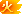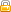[复制链接]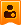楼主| 发表于 2013-5-2 09:22:29 | 显示全部楼层
 8 标注删除 [pcode=lisp,true](defun c:DimErase ()   (prompt "\n请选取要删除的尺寸标注<退出>:")   (vl-catch-all-apply     (function (lambda (/ ss)                 (if (setq ss (ssget '((0 . "DIMENSION"))))                   (command "._erase" ss "")                 )               )     )   )   (princ) )[/pcode]

### 评分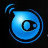XDSoft + 10 + 1 资料分享奖！楼主| 发表于 2013-5-2 09:24:12 | 显示全部楼层
 9 标注文字翻转 [pcode=lisp,true](defun c:DIMTxtRev ()   (command ".undo" "g")   (vl-catch-all-apply     (function       (lambda (/ ss ang ssl e lst)         (while (progn                  (prompt "\n请选取要翻转文字方向的尺寸标注 <退出>: ")                  (setq ss (ssget '((0 . "DIMENSION"))))                )           (setq ssl (sslength ss))           (while (> ssl 0)             (setq e   (ssname ss (setq ssl (1- ssl)))                   lst (entget e)                   ang (cdr (assoc 51 lst))             )             (entmod (subst (cons 51                                  (if (and                                        (>= pi ang)                                        (> ang 0)                                      )                                    0                                    pi                                  )                            )                            (assoc 51 lst)                            lst                     )             )           )         )       )     )   )   (command "undo" "end")   (princ) )[/pcode]楼主| 发表于 2013-5-2 09:29:54 | 显示全部楼层
 10 修改填充角度[pcode=lisp,true];;修改Hatch的角度 (defun c:ModHang (/  e el an)   (if (setq an (getangle "\n指定角度: "))     (progn       (setq el (entget e))       (entdel e)       (entmake (subst (cons 52 an)                       (assoc 52 el)                       el                )       )     )   )   (princ) )[/pcode]已领礼包: 86个财富等级: 招财进宝发表于 2013-5-2 15:49:18 | 显示全部楼层
 感谢楼主分享楼主| 发表于 2013-5-2 16:58:32 | 显示全部楼层
 11 局部重生成 [pcode=lisp,true](defun c:RegenObj (/ thisdrawing)   (setq thisdrawing (vla-get-activedocument (vlax-get-acad-object)))    (vl-catch-all-apply     (function       (lambda (/ ss)         (if (setq ss (ssget))           (progn             (vlax-for obj (vla-get-activeselectionset thisdrawing)               (vla-update obj)             )           )         )       )     )   )   (princ) )[/pcode]楼主| 发表于 2013-5-2 17:00:09 | 显示全部楼层
 12 临时隐藏[pcode=lisp,true](defun c:TmpHide ()   (vl-catch-all-apply     (function       (lambda (/ ss sl n)         (if (setq ss (ssget))           (progn             (setq sl (sslength ss)                   n  -1             )             (while (< n (1- sl))               (redraw (ssname ss (setq n (1+ n))) 2)             )           )         )       )     )   )   (princ) )[/pcode]楼主| 发表于 2013-5-2 17:02:41 | 显示全部楼层
 本帖最后由 Free-Lancer 于 2013-5-2 17:06 编辑 13 循环修改文字示例，可以是块中的文字、属性、多行文字[pcode=lisp,true] ;| 循环修改文字示例，可以是块中的文字、属性、多行文字 |; (defun c:ChgTxt ()   (vl-catch-all-apply     (function       (lambda (/ e vla-object)         (while (and (setq e (nentsel "\n选择文字<退出>: "))                     (setq vla-object                            (vlax-ename->vla-object (car e))                     )                     (member (vla-get-objectname vla-object)                             '("AcDbText" "AcDbAttribute" "AcDbMtext")                     )                )           (princ (strcat "\n原文字: "                          (vla-get-textstring vla-object)                  )           )           (vla-put-textstring             vla-object             (getstring "\n新文字: ")           )           (if (> (length e) 2)             (vla-update               (vlax-ename->vla-object (last (last e)))             )           )         )       )     )   )   (princ) )[/pcode]楼主| 发表于 2013-5-2 17:05:16 | 显示全部楼层
 14 修改点选段多义线宽度[pcode=lisp,true](defun c:Plw (/ ss e e0 p w pam p1 p2)   (while (and (setq e (entsel "\n选择多线: "))               (setq e0 (car e)                     p  (cadr e)               )               (progn                 (setq ss (ssadd))                 (ssadd e0 ss)                 (sssetfirst ss ss)                 T               )               (if                 (setq                   w (getreal                       (strcat "\n输入宽度:<"                               (vl-princ-to-string (getvar "plinewid"))                               ">: "                       )                     )                 )                  (setvar "plinewid" w)                  (setq w (getvar "plinewid"))               )          )     (setq ss (ssadd))     (ssadd e0 ss)     (sssetfirst ss ss)     (setq pam (vlax-curve-getparamatpoint                 e0                 (vlax-curve-getclosestpointto e0 p)               )           p1  (fix pam)           p2  (1+ pam)     )     (vla-setwidth (vlax-ename->vla-object e0) p1 w w)   )   (sssetfirst ss nil)   (princ) )[/pcode]楼主| 发表于 2013-5-2 17:13:54 | 显示全部楼层
 本帖最后由 Free-Lancer 于 2013-5-2 17:20 编辑 15 对齐标注转转角标注[sell=5][pcode=lisp,true](defun c:datol (/ ms doc)   (setq        doc (vla-get-activedocument (vlax-get-acad-object))         ms  (vla-get-modelspace doc)   )   (vla-startundomark doc)   (vl-catch-all-apply     '(lambda (/ ss p1 p2 an dl)        (if (setq ss (ssget "x" '((0 . "dimension"))))          (progn            (vlax-for obj (vla-get-activeselectionset doc)              (if (= (vla-get-objectname obj) "AcDbAlignedDimension")                (progn                  (setq p1 (vla-get-ExtLine1Point obj)                        p2 (vla-get-extline2point obj)                        an (angle (safearray-value (variant-value p1))                                  (safearray-value (variant-value p2))                           )                  )                  (setq dl                         (vla-adddimrotated                           ms                           p1                           p2                           (vlax-3d-point                             (cdr                               (assoc 10 (entget (vlax-vla-object->ename obj)))                             )                           )                           an                         )                  )                  (vla-put-textoverride dl (vla-get-textoverride obj))                  (vla-put-textrotation dl (vla-get-textrotation obj))                  (vla-put-textprefix dl (vla-get-textprefix obj))                  (vla-put-textsuffix dl (vla-get-textsuffix obj))                  (vla-put-textposition dl (vla-get-textposition obj))                  (vla-delete obj)                )              )            )          )        )      )   )   (vla-endundomark doc)   (princ) )[/pcode][/sell]

### 点评楼主| 发表于 2013-5-2 17:32:30 | 显示全部楼层
 16 简单的双向Offset [pcode=lisp,true](defun c:Rd ()    (vl-catch-all-apply     (function       (lambda (/ d)         (setq d (getdist "\nInput Road Width = "))         (while  (ssget)           (vlax-for obj        (vla-get-activeselectionset (vla-get-activedocument (vlax-get-acad-object)))             (vla-offset obj d)             (vla-offset obj (- d))           )         )       )     )   )   (princ) )[/pcode]

### 点评楼主| 发表于 2013-5-2 17:44:45 | 显示全部楼层
 18 用引线和字段标准Pline面积及周长[sell=5][pcode=lisp,true](defun C:FA (/ acsp adoc cpt elist en ent fld lead_obj lpt mtx oid osm)   (vl-load-com)   (or adoc       (setq adoc              (vla-get-activedocument                (vlax-get-acad-object)              )       )   )   (if (and         (= (getvar "tilemode") 0)         (= (getvar "cvport") 1)       )     (setq acsp (vla-get-paperspace adoc))     (setq acsp (vla-get-modelspace adoc))   )   (setq osm (getvar "osmode"))   (setvar "osmode" 0)   (while     (setq ent (entsel "\nSelect pline or hit Enter to exit: "))      (setq en (car ent))      (if (wcmatch (cdr (assoc 0 (setq elist (entget en))))                   "*POLYLINE"          )        (progn          (setq cpt (trans (vlax-curve-getclosestpointto en (cadr ent)) 1 0)                lpt (trans (getpoint cpt "\nPick the ending point of leader: ")                           1                           0                    )          )          (setq oID (vla-get-objectid (vlax-ename->vla-object en)))          (setq fld                 (strcat                   (strcat "Area = "                           "%<\\AcObjProp Object(%<\\_ObjId "                           (itoa oID)                           ">%).Area \\f \"%lu2%pr2\">%"                           "\\P"                   )                   (strcat "Perimeter = "                           "%<\\AcObjProp Object(%<\\_ObjId "                           (itoa oID)                           ">%).Length \\f \"%lu2%pr2\">%"                   )                 )          )          (setq mtx (vlax-invoke                      acsp 'AddMText lpt        0.0 fld)          )          (vlax-put mtx                    'AttachmentPoint                    (cond ((> (car cpt) (car lpt))                           6                          )                          ((< (car cpt) (car lpt))                           4                          )                          (T 4)                    )          )          (vlax-put mtx 'Height (getvar "textsize"))          (setq lead_obj        (vlax-invoke                           acsp                           'Addleader                           (apply 'append (list cpt lpt))                           mtx                           acLineWithArrow                         )          )          (vlax-put lead_obj 'VerticalTextPosition 0) ;1        )      )   )   (setvar "osmode" osm)   (princ) ) (princ "\n Start command with FA ...") (princ)[/pcode][/sell]楼主| 发表于 2013-5-2 17:48:34 | 显示全部楼层
 18 统一改字体[pcode=lisp,true](defun c:stgb (/ table)   ;;===================   (defun table (s / d r)     (while (setq d (tblnext s (null d)))       (setq r (cons (cdr (assoc 2 d)) r))     )     (reverse r)   )   (setvar "cmdecho" 0)   (foreach x (table "style")     (vl-cmdf ".style" x        "gbenor,gbcbig"        "0.00" "1.0" "0.0" "n" "n")   )   (princ) )[/pcode]

### 点评

Free-Lancer ：“18 统一改字体”的程序好像是双向偏移吧，并非是改字体！  发表于 2013-7-4 23:13楼主| 发表于 2013-5-2 17:54:02 | 显示全部楼层
 19 简单的文字排版[pcode=lisp,true](defun c:tt (/ ss ssl i e el ll d hi p vh vx vy str)   (if (setq ss (ssget '((0 . "text"))))     (progn       (setq ssl        (sslength ss)             i        -1       )       (repeat ssl         (setq e         (ssname ss (setq i (1+ i)))               el (entget e)         )         (if ll           (setq ll (cons el ll))           (setq ll (list el))         )       )       (setq         ll (vl-sort ll                     '(lambda (e1 e2)                        (> (caddr (assoc 10 e1)) (caddr (assoc 10 e2)))                      )            )       )       (setq p  (cdr (assoc 10 (car ll)))             vx (car p)             vy (cadr p)             i  1       )       (setq d (getdist p "\n行距: "))       (setq hi (getdist        p                         (strcat        "\n字高<"                                 (rtos (cdr (assoc 40 (car ll)))                                       2                                       2                                 )                                 ">: "                         )                )       )       (if hi         (setq vh (cons 40 hi))       )       (foreach x ll         (if vh           (entmod (subst vh (assoc 40 x) x))         )         (setq str (vl-string-left-trim " " (cdr (assoc 1 x))))         (if (wcmatch str "[0-9]*")           (setq        str (vl-string-left-trim "1234567890" str)                 el  (subst (cons 1 (strcat (itoa i) str)) (assoc 1 x) x)                 i (1+ i)           )           (setq el x)         )         (setq el (subst (list 10 vx vy 0.) (assoc 10 x) el))         (entmod el)         (if d           (setq vy (- vy d))         )               )     )   )   (princ) )[/pcode]已领礼包: 6466个财富等级: 富甲天下发表于 2013-5-3 01:58:02 | 显示全部楼层
 谢谢楼主放出这么多好程式。已领礼包: 5563个财富等级: 富甲天下发表于 2013-5-3 08:51:40 | 显示全部楼层
 楼主辛苦！谢谢楼主的好程序！

 本版积分规则 回帖并转播 回帖后跳转到最后一页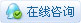|申请友链|小黑屋|手机版|Archiver|辽公网安备|晓东CAD家园 ( 辽ICP备15016793号 )

GMT+8, 2021-7-28 09:44 , Processed in 0.788160 second(s), 55 queries , Gzip On.